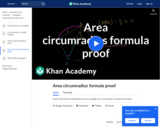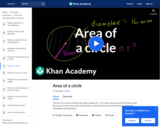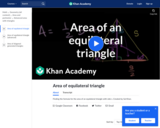Updating search results...

# 196 Results

View
Selected filters:
• GeometryConditional Remix & Share Permitted
CC BY-NC-SA
Rating
0.0 stars

This video is from the Khan Academy subject of Math on the topic of Geometry and it covers Algebraic midpoint of a segment exercise.

Subject:
Geometry
Mathematics
Material Type:
Lesson
Provider:
Author:
09/22/2013Educational Use
Rating
0.0 stars

Students learn about linear programming (also called linear optimization) to solve engineering design problems. As they work through a word problem as a class, they learn about the ideas of constraints, feasibility and optimization related to graphing linear equalities. Then they apply this information to solve two practice engineering design problems related to optimizing materials and cost by graphing inequalities, determining coordinates and equations from their graphs, and solving their equations. It is suggested that students conduct the associated activity, Optimizing Pencils in a Tray, before this lesson, although either order is acceptable.

Subject:
Algebra
Geometry
Mathematics
Material Type:
Lesson
Provider:
TeachEngineering
Provider Set:
TeachEngineering
Author:
Andi Vicksman
Malinda Zarske
Nathan Coyle
Russell Anderson
Ryan Sullivan
09/15/2020Conditional Remix & Share Permitted
CC BY-NC-SA
Rating
0.0 stars

Using the angle bisector theorem to solve for sides of a triangle

Subject:
Geometry
Mathematics
Material Type:
Lesson
Provider:
Author:
Sal Khan
09/22/2013Conditional Remix & Share Permitted
CC BY-NC-SA
Rating
0.0 stars

What the angle bisector theorem is and its proof

Subject:
Geometry
Mathematics
Material Type:
Lesson
Provider:
Author:
Sal Khan
09/22/2013Conditional Remix & Share Permitted
CC BY-NC-SA
Rating
0.0 stars

Using what we know to solve for angles in the Angle Game.

Subject:
Geometry
Mathematics
Material Type:
Lesson
Provider:
Author:
Sal Khan
09/22/2013Conditional Remix & Share Permitted
CC BY-NC-SA
Rating
0.0 stars

Definition of an angle. How to denote an angle using points on the angle (including the vertex)

Subject:
Geometry
Mathematics
Material Type:
Lesson
Provider:
Author:
Sal Khan
09/22/2013Conditional Remix & Share Permitted
CC BY-NC-SA
Rating
0.0 stars

Parallel lines, transversal lines, corresponding angles

Subject:
Geometry
Mathematics
Material Type:
Lesson
Provider:
Author:
Sal Khan
09/22/2013Conditional Remix & Share Permitted
CC BY-NC-SA
Rating
0.0 stars

Angles of parallel lines

Subject:
Geometry
Mathematics
Material Type:
Lesson
Provider:
Author:
Sal Khan
09/22/2013Conditional Remix & Share Permitted
CC BY-NC-SA
Rating
0.0 stars

Angles of parallel lines examples

Subject:
Geometry
Mathematics
Material Type:
Lesson
Provider:
Author:
Sal Khan
09/22/2013Conditional Remix & Share Permitted
CC BY-NC-SA
Rating
0.0 stars

Possible angles for an isosceles triangle

Subject:
Geometry
Mathematics
Material Type:
Lesson
Provider:
Author:
Sal Khan
04/14/2016Conditional Remix & Share Permitted
CC BY-NC-SA
Rating
0.0 stars

Visually proving the Pythagorean Theorem

Subject:
Geometry
Mathematics
Material Type:
Lesson
Provider:
Author:
Sal Khan
09/22/2013Conditional Remix & Share Permitted
CC BY-NC-SA
Rating
0.0 stars

Proof of the formula relating the area of a triangle to its circumradius

Subject:
Geometry
Mathematics
Material Type:
Lesson
Provider:
Author:
MIT+K12
09/22/2013Conditional Remix & Share Permitted
CC BY-NC-SA
Rating
0.0 stars

Area of Diagonal Generated Triangles of Rectangle are Equal

Subject:
Geometry
Mathematics
Material Type:
Lesson
Provider:
Author:
09/22/2013Conditional Remix & Share Permitted
CC BY-NC-SA
Rating
0.0 stars

Area of a Circle

Subject:
Geometry
Mathematics
Material Type:
Lesson
Provider:
Author:
Sal Khan
04/14/2016Conditional Remix & Share Permitted
CC BY-NC-SA
Rating
0.0 stars

Showing that the area of a parallelogram is base times height

Subject:
Geometry
Mathematics
Material Type:
Lesson
Provider:
Author:
09/22/2013Conditional Remix & Share Permitted
CC BY-NC-SA
Rating
0.0 stars

Using what we know about triangles to find the area of a regular hexagon

Subject:
Geometry
Mathematics
Material Type:
Lesson
Provider:
Author:
Sal Khan
09/22/2013Conditional Remix & Share Permitted
CC BY-NC-SA
Rating
0.0 stars

This video is from the Khan Academy subject of Math on the topic of Geometry and it covers Area of a kite.

Subject:
Geometry
Mathematics
Material Type:
Lesson
Provider:
Author:
11/16/2016Conditional Remix & Share Permitted
CC BY-NC-SA
Rating
0.0 stars

Finding the formula for the area of an equilateral triangle with side s

Subject:
Geometry
Mathematics
Material Type:
Lesson
Provider:
Author:
Sal Khan
09/22/2013Conditional Remix & Share Permitted
CC BY-NC-SA
Rating
0.0 stars

This video is from the Khan Academy subject of Math on the topic of Geometry and it covers Area of a sector given a central angle.

Subject:
Geometry
Mathematics
Material Type:
Lesson
Provider:
Author:
Sal Khan
09/22/2013Conditional Remix & Share Permitted
CC BY-NC-SA
Rating
0.0 stars

This video is from the Khan Academy subject of Math on the topic of Geometry and it covers Area of a trapezoid.

Subject:
Geometry
Mathematics
Material Type:
Lesson
Provider: